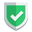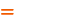我们相信：世界是美好的，你是我也是。平行空间的世界里面，不同版本的生活也在继续...

## 基本定义

``````import pandas as pd
from pandas import Series, DataFrame
df = DataFrame([
('虎子', 5, "dog", "5kg", True),
('老许', 3, "bird", "3kg", True),
('二赖子', 6, "fish", "6kg", True),
('老白', 8, "catty", "5kg", True),
('小黑', 10, "puppy", "2kg", True),
],
columns=('name', 'age', 'class', 'weight', 'good')
)
# df_copy = df.copy()``````

• `inplace=False``inplace`默认为`False`，意思是不改变原数据。改变后的数据会被返回。
• `inplace=True`，这种情况下，没有返回值。直接修改原数据。

## 删除一列数据

### 方式一`del`

``del df['good']``

``````del df['good']
print(df)``````
``````  name  age  class weight
0   虎子    5    dog    5kg
1   老许    3   bird    3kg
2  二赖子    6   fish    6kg
3   老白    8  catty    5kg
4   小黑   10  puppy    2kg``````

### 方式二`.pop()`

``_series = df.pop("good")``

``````a = df.pop('weight')
print(a)``````
``````0    5kg
1    3kg
2    6kg
3    5kg
4    2kg
Name: weight, dtype: object``````

### 方式三`.drop('name',axis=1)`

``````df2 = df.drop('age',axis=1)
df2 = df.drop(['age'],axis=1)
df2 = df.drop(['age', 'class'],axis=1)``````
``````df.drop('age',axis=1,inplace=True)
df.drop(['age'],axis=1,inplace=True)
df.drop(['age', 'class'],axis=1,inplace=True)``````

``````df2 = df.drop('class',axis=1,inplace=False)
print(df)
print(df2)``````
``````  name  age  class weight  good
0   虎子    5    dog    5kg  True
1   老许    3   bird    3kg  True
2  二赖子    6   fish    6kg  True
3   老白    8  catty    5kg  True
4   小黑   10  puppy    2kg  True
name  age weight  good
0   虎子    5    5kg  True
1   老许    3    3kg  True
2  二赖子    6    6kg  True
3   老白    8    5kg  True
4   小黑   10    2kg  True``````

``````df3 = df.drop('class',axis=1,inplace=True)
print(df)
print(df3)``````
``````  name  age weight  good
0   虎子    5    5kg  True
1   老许    3    3kg  True
2  二赖子    6    6kg  True
3   老白    8    5kg  True
4   小黑   10    2kg  True
None``````

### 方式四`.drop(columns=['weight', 'good'])`

`axis=1`的意思就是`columns`，意思是删除一列数据。这里，同样存在`inplace`的问题。

``````df2 = df.drop(columns=['weight'])
df2 = df.drop(columns=['weight', 'good'])
df2 = df.drop(columns=['weight', 'good'])``````
``````df.drop(columns=['weight'], inplace=True)
df.drop(columns=['weight', 'good'], inplace=True)
df.drop(columns=['weight', 'good'], inplace=True)``````

``````bb = df.drop(columns=['weight', 'good'])
print(df)
print(bb)``````
``````  name  age  class weight  good
0   虎子    5    dog    5kg  True
1   老许    3   bird    3kg  True
2  二赖子    6   fish    6kg  True
3   老白    8  catty    5kg  True
4   小黑   10  puppy    2kg  True
name  age  class
0   虎子    5    dog
1   老许    3   bird
2  二赖子    6   fish
3   老白    8  catty
4   小黑   10  puppy``````

## 删除一行数据

``````df2 = df.drop(0)
df2 = df.drop([0,1,2,3])
df2 = df.drop(index=[0,1,2,3])``````
``````df.drop(0, inplace=True)
df.drop([0,1,2,3], inplace=True)
df.drop(index=[0,1,2,3], inplace=True)``````

``````print(df)
df2 = df.drop(1)
df3 = df.drop([0, 1])
print(df2)
print(df3)``````
``````  name  age  class weight  good
0   虎子    5    dog    5kg  True
1   老许    3   bird    3kg  True
2  二赖子    6   fish    6kg  True
3   老白    8  catty    5kg  True
4   小黑   10  puppy    2kg  True
name  age  class weight  good
0   虎子    5    dog    5kg  True
2  二赖子    6   fish    6kg  True
3   老白    8  catty    5kg  True
4   小黑   10  puppy    2kg  True
name  age  class weight  good
2  二赖子    6   fish    6kg  True
3   老白    8  catty    5kg  True
4   小黑   10  puppy    2kg  True``````
``````df4 = df.drop(index=[0, 1], inplace=True)
print(df)
print(df4)``````
``````  name  age  class weight  good
2  二赖子    6   fish    6kg  True
3   老白    8  catty    5kg  True
4   小黑   10  puppy    2kg  True
None``````

## 总结

`dataframe`的删除数据的方式似乎有点多，虽然每个函数都有它存在的理由，不过这里苏南大叔觉得这些函数太多了。头晕。如果本文对您有帮助，或者节约了您的时间，欢迎打赏瓶饮料，建立下友谊关系。本博客不欢迎：各种镜像采集行为。请尊重原创文章内容，转载请保留作者链接。【福利】 腾讯云最新爆款活动！1核2G云服务器首年50元！【源码】本文代码片段及相关软件，请点此获取更多信息【绝密】秘籍文章入口，仅传授于有缘之人pandas